3.2. Age of the universe and cosmological constant

From equation (24) we can also determine the current age of the universe by the integral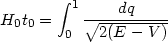(34)

Since most of the contribution to this integral comes from late times, we can ignore the radiation term and setR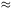0. When bothNR and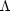are present and are arbitrary, the age of the universe is determined by the integral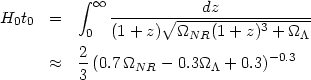(35)

The integral, which cannot be expressed in terms of elementary functions, is well approximated by the numerical fit given in the second line. Contours of constant H0t0 based on the (exact) integral are shown in figure 11. It is obvious that, for a givenNR, the age is higher for models with0.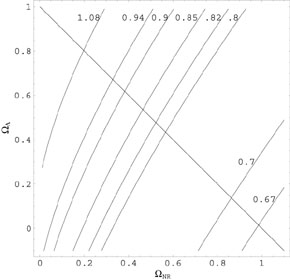Figure 11. Lines of constant H0t0 in theNR -plane. The eight lines are for H0 t0 = (1.08, 0.94, 0.9, 0.85, 0.82, 0.8, 0.7, 0.67) as shown. The diagonal line is the contour for models withNR += 1.

Observationally, there is a consensus [49, 50] that h0.72 ± 0.07 and t013.5 ± 1.5 Gyr . This will give H0 t0 = 0.94 ± 0.14. Comparing this result with the fit in (35), one can immediately draw several conclusions:

• IfNR > 0.1, thenis non zero if H0 t0 > 0.9. A more reasonable assumption ofNR > 0.3 we will require non zeroif H0 t0 > 0.82.
• If we takeNR = 1,= 0 and demand t0 > 12 Gyr (which is a conservative lower bound from stellar ages) will require h < 0.54. Thus a purely matter dominated= 1 universe would require low Hubble constant which is contradicted by most of the observations.
• An open model withNR0.2,= 0 will require H0 t00.85. This still requires ages on the lower side but values like h0.6, t013.5 Gyr are acceptable within error bars.
• A straightforward interpretation of observations suggests maximum likelihood for H0 t0 = 0.94. This can be consistent with a= 1 model only ifNR0.3,0.7.

If the universe is populated by dust-like matter (with w = 0) and another component with an equation of state parameter wX, then the age of the universe will again be given by an integral similar to the one in equation (35) withreplaced byX(1 + z)3(1+wX). This will give(36)

The integrand varies from 0 to (NR +X)-1/2 in the range of integration for w < 0 with the rapidity of variation decided by w. As a result, H0 t0 increases rapidly as w changes from 0 to -3 or so and then saturates to a plateau. Even an absurdly negative value for w like w = - 100 gives H0 t0 of only about 1.48. This shows that even if some exotic dark energy is present in the universe with a constant, negative w, it cannot increase the age of the universe beyond about H0 t01.48.

The comments made above pertain to the current age of the universe. It is also possible to obtain an expression similar to (34) for the age of the universe at any given redshift z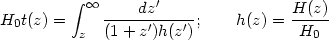(37)

and use it to constrain. For example, the existence of high redshift galaxies with evolved stellar population, high redshift radio galaxies and age dating of high redshift QSOs can all be used in conjunction with this equation to put constrains on[84, 85, 86, 87, 88, 89]. Most of these observations require either0 ortot < 1 if they have to be consistent with h0.6. Unfortunately, the interpretation of these observations at present requires fairly complex modeling and hence the results are not water tight.